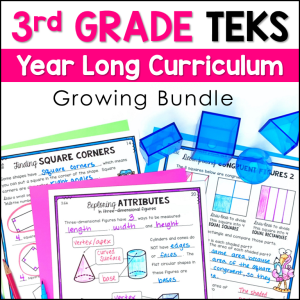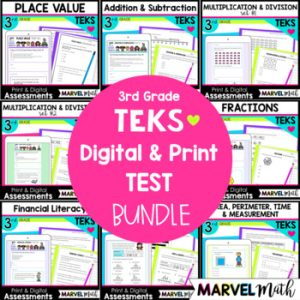Save 10% on your first order with code EXTRA10

# 3rd Grade Fraction Tests – Digital and Print

\$4.00\$151.25\$18.00
SKU: 7200443 Categories: ,

## Description

This resource includes 2 ten question Assessments that cover the Fractions TEKS. The questions are STAAR formatted. Test questions cover fractions on a number line, equivalent fractions, comparing fractions, and reasoning about fractions. The assessments are in 2 formats: printable PDF and digital, self-grading Google Forms.

The assessments are created to give you meaningful feedback on student progress with Fractions Content. There are more questions covering the Readiness Standards than the Supporting Standards, as the Readiness Standards are identified as the most critical content for success in subsequent grade levels. Readiness Standards also comprise 2/3 of the STAAR test.

These tests were created with the new STAAR test questions guidelines in mind, and include drop down, multi-select, free response and multiple choice questions.

What’s Included?

• 2 Assessments (Each Test is 10 questions)
• Printable versions of both Assessments
• Color Coded Teacher Key with TEKS identified for each Question

Topics include:

• Representing Fractions with Models
• Fractions on a Number Line
• Explaining Unit Fractions
• Representing Equivalent Fractions with Models and on Number Lines
• Comparing Fractions
• Composing and Decomposing Fractions into unit fractions (3/4 = 1/4 + 1/4 + 1/4)
• Dividing Objects into Fractional Shares

These tests coordinate with the content covered in this set of Task Cards and these resources work well together!

How Can I Use this Resource?

• Use the 1st test after your Fractions Unit. Based on the results, do targeted reteach and review. Use the 2nd test to re-assess students.
• Use the 1st test after your Fractions Unit. Use the 2nd test as part of your STAAR Review.
• Use the 1st test as a pre-test, use the 2nd test as a post-test.

TEKS Alignment:

3.3F: Represent equivalent fractions with denominators of 2, 3, 4, 6, and 8 using a variety of objects and pictorial models, including number lines.

3.3H: Compare two fractions having the same numerator or denominator in problems by reasoning about their sizes and justifying the conclusion using symbols, words, objects, and pictorial models.

Supporting Standards

3.3A: Represent fractions greater than zero and less than or equal to one with denominators of 2, 3, 4, 6, and 8 using concrete objects and pictorial models, including strip diagrams and number lines.

3.3B: Determine the corresponding fraction greater than zero and less than or equal to one with denominators of 2, 3, 4, 6, and 8 given a specified point on a number line.

3.3C: Explain that the unit fraction 1/b represents the quantity formed by one part of a whole that has been partitioned into b equal parts where b is a non-zero whole number.

3.3D: Compose and decompose a fraction a/b with a numerator greater than zero and less than or equal to b as a sum of parts 1/b.

3.3E: Solve problems involving partitioning an object or a set of objects among two or more recipients using pictorial representations of fractions with denominators of 2, 3, 4, 6, and 8.

3.3G: Explain that two fractions are equivalent if and only if they are both represented by the same point on the number line or represent the same portion of a same size whole for an area model.